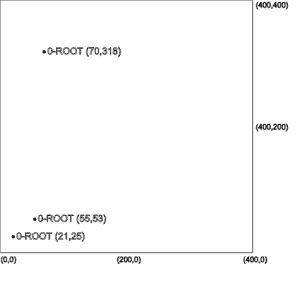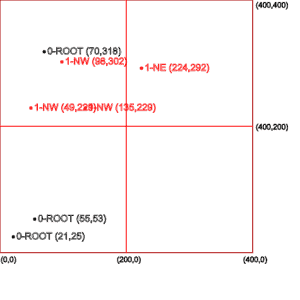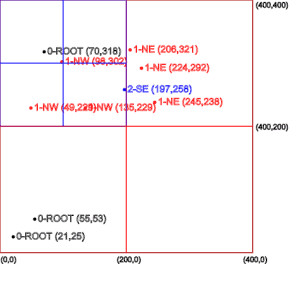## 1. Overview

In this tutorial, we’ll explore the concept of searching for neighbors in a two-dimensional space. Then, we’ll walk through its implementation in Java.

We know that binary search is an efficient algorithm for finding an exact match in a list of items using a divide-and-conquer approach.

Let’s now consider a two-dimensional area where each item is represented by XY coordinates (points) in a plane.

However, instead of an exact match, suppose we want to find neighbors of a given point in the plane. It’s clear that if we want the nearest n matches, then the binary search will not work. This is because the binary search can compare two items in one axis only, whereas we need to be able to compare them in two axes.

We’ll look at an alternative to the binary tree data structure in the next section.

A quadtree is a spatial tree data structure in which each node has exactly four children. Each child can either be a point or a list containing four sub-quadtrees.

A point stores data — for example, XY coordinates. A region represents a closed boundary within which a point can be stored. It is used to define the area of reach of a quadtree.

Let’s understand this more using an example of 10 coordinates in some arbitrary order:

``(21,25), (55,53), (70,318), (98,302), (49,229), (135,229), (224,292), (206,321), (197,258), (245,238)``

The first three values will be stored as points under the root node as shown in the left-most picture.The root node cannot accommodate new points now as it has reached its capacity of three points. Therefore, we’ll divide the region of the root node into four equal quadrants.

Each of these quadrants can store three points and additionally contain four quadrants within its boundary. This can be done recursively, resulting in a tree of quadrants, which is where the quadtree data structure gets its name.

In the middle picture above, we can see the quadrants created from the root node and how the next four points are stored in these quadrants.

Finally, the right-most picture shows how one quadrant is again subdivided to accommodate more points in that region while the other quadrants still can accept the new points.

We’ll now see how to implement this algorithm in Java.

## 4. Data Structure

Let’s create a quadtree data structure. We’ll need three domain classes.

Firstly, we’ll create a Point class to store the XY coordinates:

``````public class Point {
private float x;
private float y;

public Point(float x, float y) {
this.x = x;
this.y = y;
}

// getters & toString()
}``````

Secondly, let’s create a Region class to define the boundaries of a quadrant:

``````public class Region {
private float x1;
private float y1;
private float x2;
private float y2;

public Region(float x1, float y1, float x2, float y2) {
this.x1 = x1;
this.y1 = y1;
this.x2 = x2;
this.y2 = y2;
}

// getters & toString()
}``````

Finally, let’s have a QuadTree class to store data as Point instances and children as QuadTree classes:

``````public class QuadTree {
private static final int MAX_POINTS = 3;
private Region area;
private List<Point> points = new ArrayList<>();

this.area = area;
}
}``````

To instantiate a QuadTree object, we specify its area using the Region class through the constructor.

## 5. Algorithm

Before we write our core logic to store data, let’s add a few helper methods. These will prove useful later.

### 5.1. Helper Methods

Let’s modify our Region class.

Firstly, let’s have a method containsPoint to indicate if a given point falls inside or outside of a region’s area:

``````public boolean containsPoint(Point point) {
return point.getX() >= this.x1
&& point.getX() < this.x2
&& point.getY() >= this.y1
&& point.getY() < this.y2;
}``````

Next, let’s have a method doesOverlap to indicate if a given region overlaps with another region:

``````public boolean doesOverlap(Region testRegion) {
if (testRegion.getX2() < this.getX1()) {
return false;
}
if (testRegion.getX1() > this.getX2()) {
return false;
}
if (testRegion.getY1() > this.getY2()) {
return false;
}
if (testRegion.getY2() < this.getY1()) {
return false;
}
return true;
}``````

Finally, let’s create a method getQuadrant to divide a range into four equal quadrants and return a specified one:

``````public Region getQuadrant(int quadrantIndex) {
float quadrantWidth = (this.x2 - this.x1) / 2;
float quadrantHeight = (this.y2 - this.y1) / 2;

// 0=SW, 1=NW, 2=NE, 3=SE
case 0:
case 1:
case 2:
case 3:
}
return null;
}``````

### 5.2. Storing Data

We can now write our logic to store data. Let’s start by defining a new method addPoint on the QuadTree class to add a new point. This method will return true if a point was successfully added:

``````public boolean addPoint(Point point) {
// ...
}``````

Next, let’s write the logic to handle the point. First, we need to check if the point is contained within the boundary of the QuadTree instance. We also need to ensure that the QuadTree instance has not reached the capacity of MAX_POINTS points.

If both the conditions are satisfied, we can add the new point:

``````if (this.area.containsPoint(point)) {
if (this.points.size() < MAX_POINTS) {
return true;
}
}``````

On the other hand, if we’ve reached the MAX_POINTS value, then we need to add the new point to one of the sub-quadrants. For this, we loop through the child quadTrees list and call the same addPoint method which will return a true value on successful addition. Then we exit the loop immediately as a point needs to be added exactly to one quadrant.

We can encapsulate all this logic inside a helper method:

``````private boolean addPointToOneQuadrant(Point point) {
for (int i = 0; i < 4; i++) {
return true;
}
return false;
}``````

``````private void createQuadrants() {
Region region;
for (int i = 0; i < 4; i++) {
}
}``````

We’ll call this method to create quadrants only if we’re no longer able to add any new points. This ensures that our data structure uses optimum memory space.

Putting it all together, we’ve got the updated addPoint method:

``````public boolean addPoint(Point point) {
if (this.area.containsPoint(point)) {
if (this.points.size() < MAX_POINTS) {
return true;
} else {
}
}
}
return false;
}``````

### 5.3. Searching Data

Having our quadtree structure defined to store data, we can now think of the logic for performing a search.

As we’re looking for finding adjacent items, we can specify a searchRegion as the starting point. Then, we check if it overlaps with the root region. If it does, then we add all its child points that fall inside the searchRegion.

After the root region, we get into each of the quadrants and repeat the process. This goes on until we reach the end of the tree.

Let’s write the above logic as a recursive method in the QuadTree class:

``````public List<Point> search(Region searchRegion, List<Point> matches) {
if (matches == null) {
matches = new ArrayList<Point>();
}
if (!this.area.doesOverlap(searchRegion)) {
return matches;
} else {
for (Point point : points) {
if (searchRegion.containsPoint(point)) {
}
}
for (int i = 0; i < 4; i++) {
.search(searchRegion, matches);
}
}
}
return matches;
}``````

## 6. Testing

Now that we have our algorithm in place, let’s test it.

### 6.1. Populating the Data

First, let’s populate the quadtree with the same 10 coordinates we used earlier:

``````Region area = new Region(0, 0, 400, 400);

float[][] points = new float[][] { { 21, 25 }, { 55, 53 }, { 70, 318 }, { 98, 302 },
{ 49, 229 }, { 135, 229 }, { 224, 292 }, { 206, 321 }, { 197, 258 }, { 245, 238 } };

for (int i = 0; i < points.length; i++) {
Point point = new Point(points[i], points[i]);
}``````

Next, let’s perform a range search in an area enclosed by lower bound coordinate (200, 200) and upper bound coordinate (250, 250):

``````Region searchArea = new Region(200, 200, 250, 250);

Running the code will give us one nearby coordinate contained within the search area:

``[[245.0 , 238.0]]``

Let’s try a different search area between coordinates (0, 0) and (100, 100):

``````Region searchArea = new Region(0, 0, 100, 100);

Running the code will give us two nearby coordinates for the specified search area:

``[[21.0 , 25.0], [55.0 , 53.0]]``

We observe that depending on the size of the search area, we get zero, one or many points. So, if we’re given a point and asked to find the nearest n neighbors, we could define a suitable search area where the given point is at the center.

Then, from all the resulting points of the search operation, we can calculate the Euclidean distances between the given points and sort them to get the nearest neighbors.

## 7. Time Complexity

The time complexity of a range query is simply O(n). The reason is that, in the worst-case scenario, it has to traverse through each item if the search area specified is equal to or bigger than the populated area.

## 8. Conclusion

In this article, we first understood the concept of a quadtree by comparing it with a binary tree. Next, we saw how it can be used efficiently to store data spread across a two-dimensional space.

We then saw how to store data and perform a range search.

As always, the source code with tests is available over on GitHub.

### Get started with Spring 5 and Spring Boot 2, through the Learn Spring course:

>> CHECK OUT THE COURSE